# Inorganic Chemistry Questions

The best high school and college tutors are just a click away, 24×7! Pick a subject, ask a question, and get a detailed, handwritten solution personalized for you in minutes. We cover Math, Physics, Chemistry & Biology.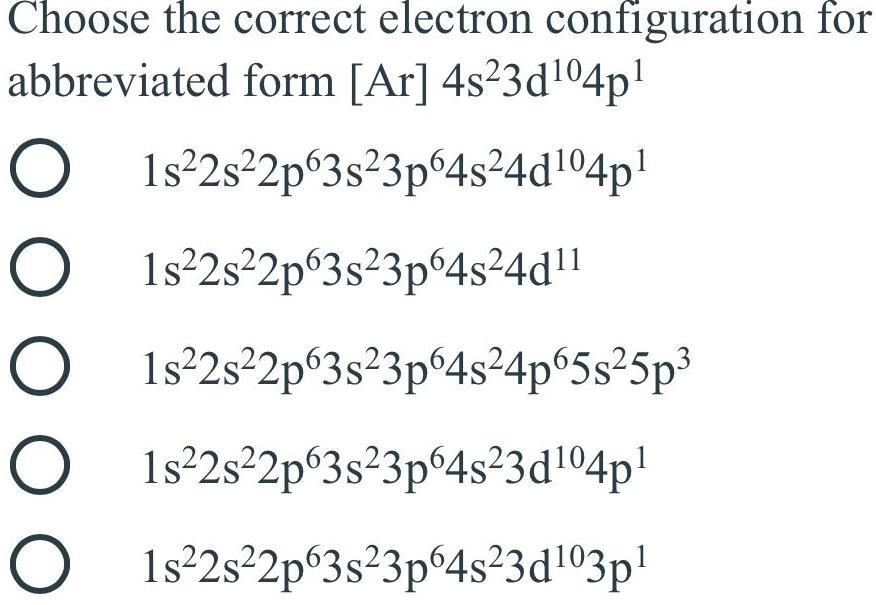Inorganic Chemistry
F Block elements
Choose the correct electron configuration for abbreviated form [Ar] 4s²3d¹04p¹ A. 1s²2s²2p 3s²3p64s²4d¹04p¹ B. 1s²2s²2p63s²3p64s²4d¹¹ C. 1s²2s²2p63s²3p64s²4p65s²5p³ D. 1s²2s²2p63s²3p64s²3d¹04p¹ E. 1s²2s²2p63s²3p64s²3d¹03p¹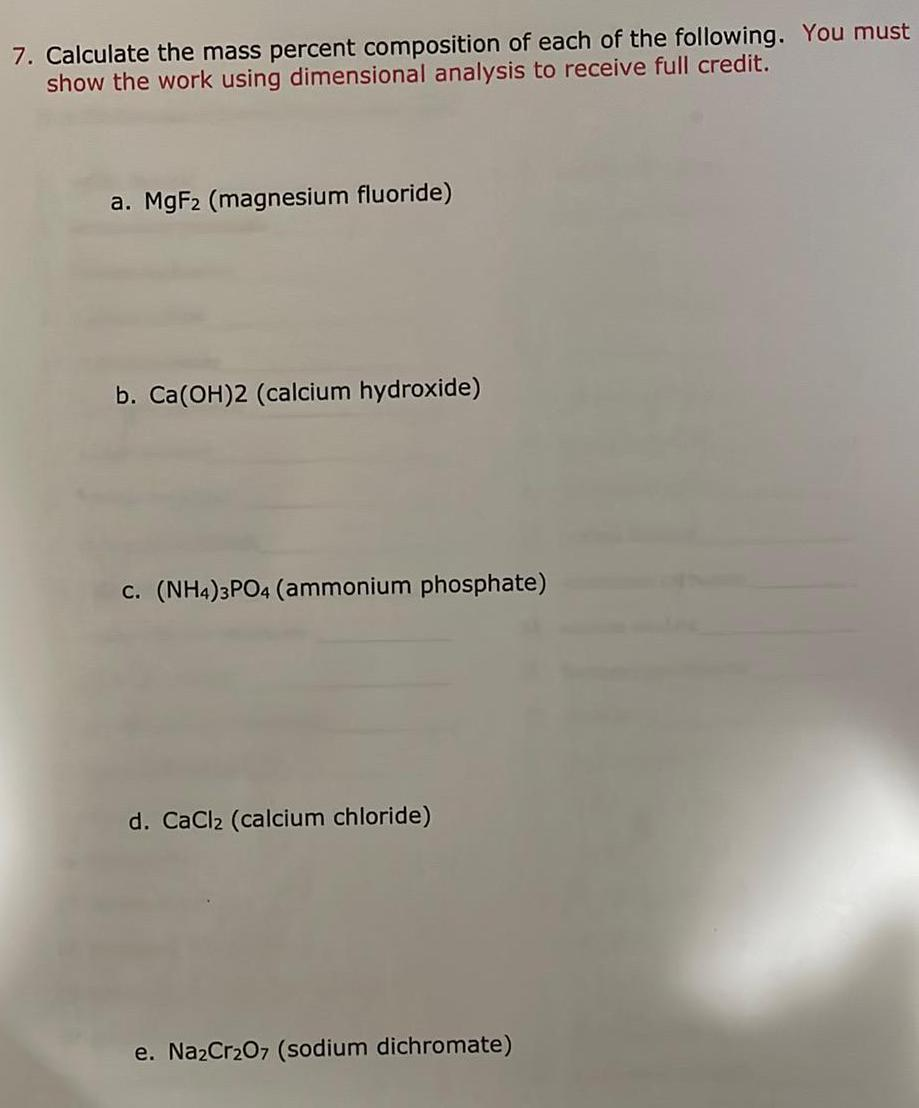Inorganic Chemistry
Preparation and Properties of Compounds
7. Calculate the mass percent composition of each of the following. a. MgF2 (magnesium fluoride) b. Ca(OH)2 (calcium hydroxide) c. (NH4)3PO4 (ammonium phosphate) d. CaCl2 (calcium chloride) e. Na2Cr₂O7 (sodium dichromate)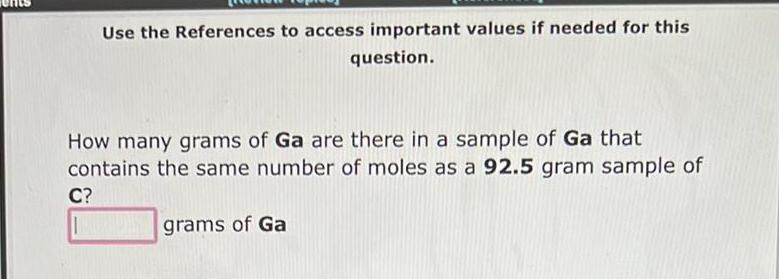Inorganic Chemistry
Preparation and Properties of Compounds
How many grams of Ga are there in a sample of Ga that contains the same number of moles as a 92.5 gram sample of C?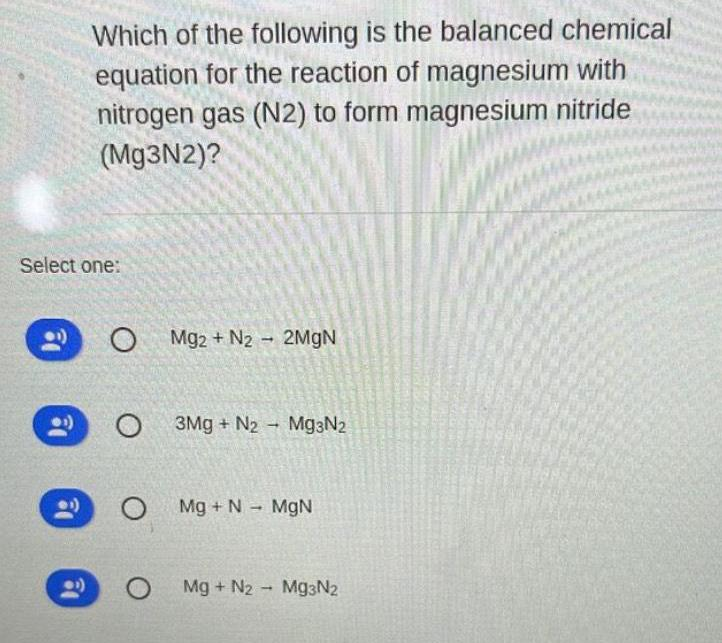Inorganic Chemistry
Preparation and Properties of Compounds
Which of the following is the balanced chemical equation for the reaction of magnesium with nitrogen gas (N2) to form magnesium nitride (Mg3N2)? Mg2+ N2 2MgN 3Mg + N2 Mg3N₂ Mg + N → MgN Mg + N2 Mg3N2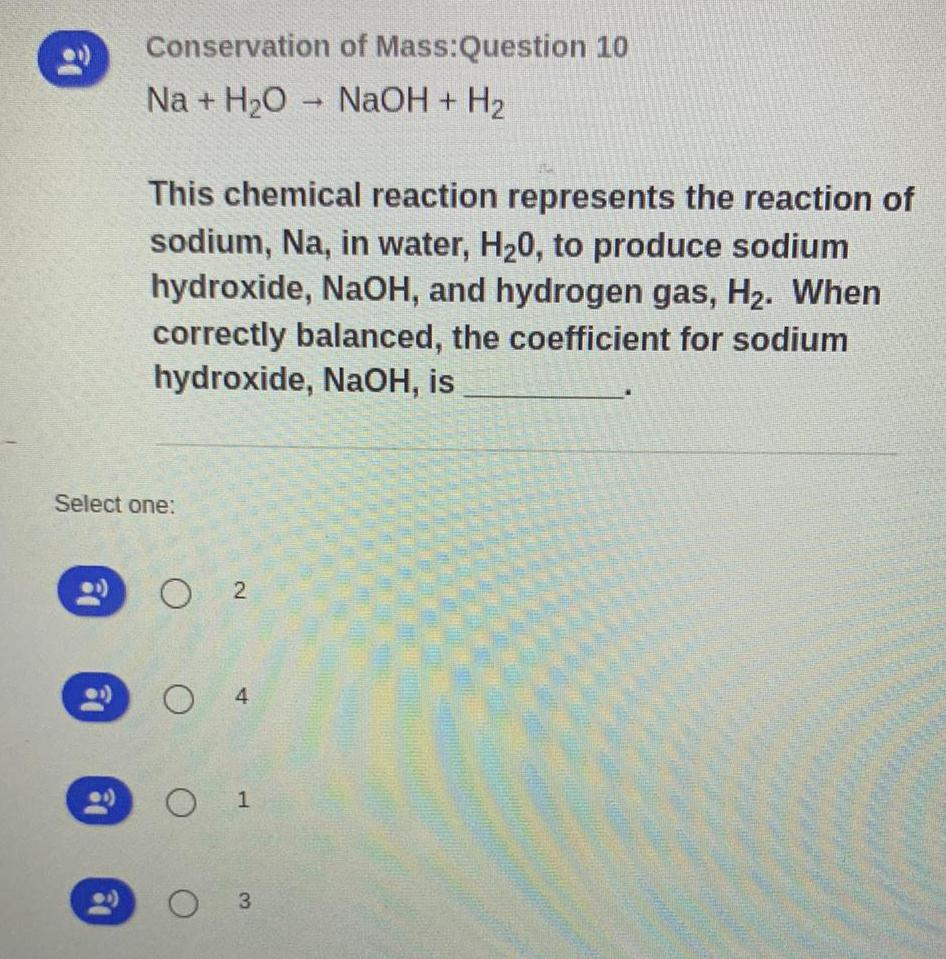Inorganic Chemistry
Classification of Elements and Periodicity
Na + H₂O → NaOH + H₂ This chemical reaction represents the reaction of sodium, Na, in water, H₂O, to produce sodium hydroxide, NaOH, and hydrogen gas, H₂. When correctly balanced, the coefficient for sodium hydroxide, NaOH, is_____ A. 2 B. 4 C. 1 D. 3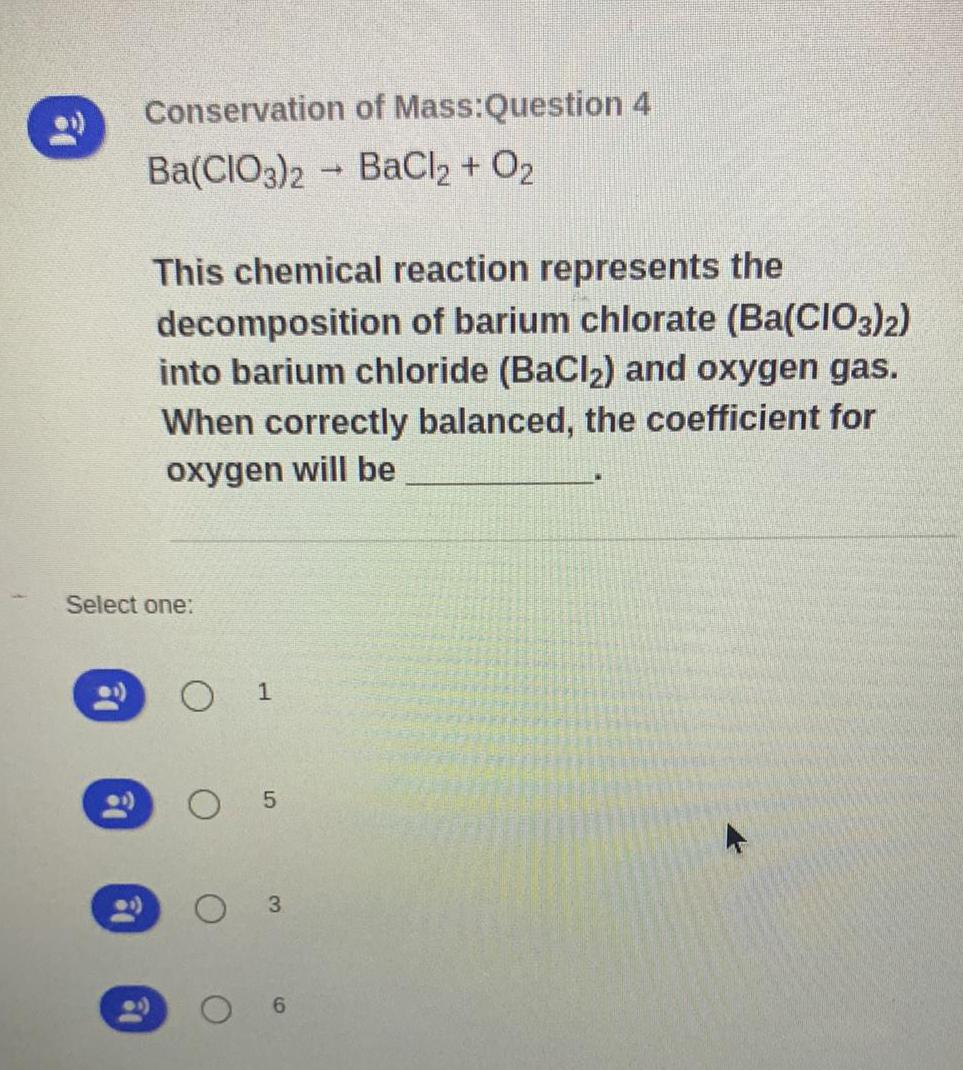Inorganic Chemistry
Classification of Elements and Periodicity
Ba(CIO3)2 BaCl2 + O₂ This chemical reaction represents the decomposition of barium chlorate (Ba(CIO3)2) into barium chloride (BaCl₂) and oxygen gas. When correctly balanced, the coefficient for oxygen will be ____ A. 1 B. 5 C. 3 D. 6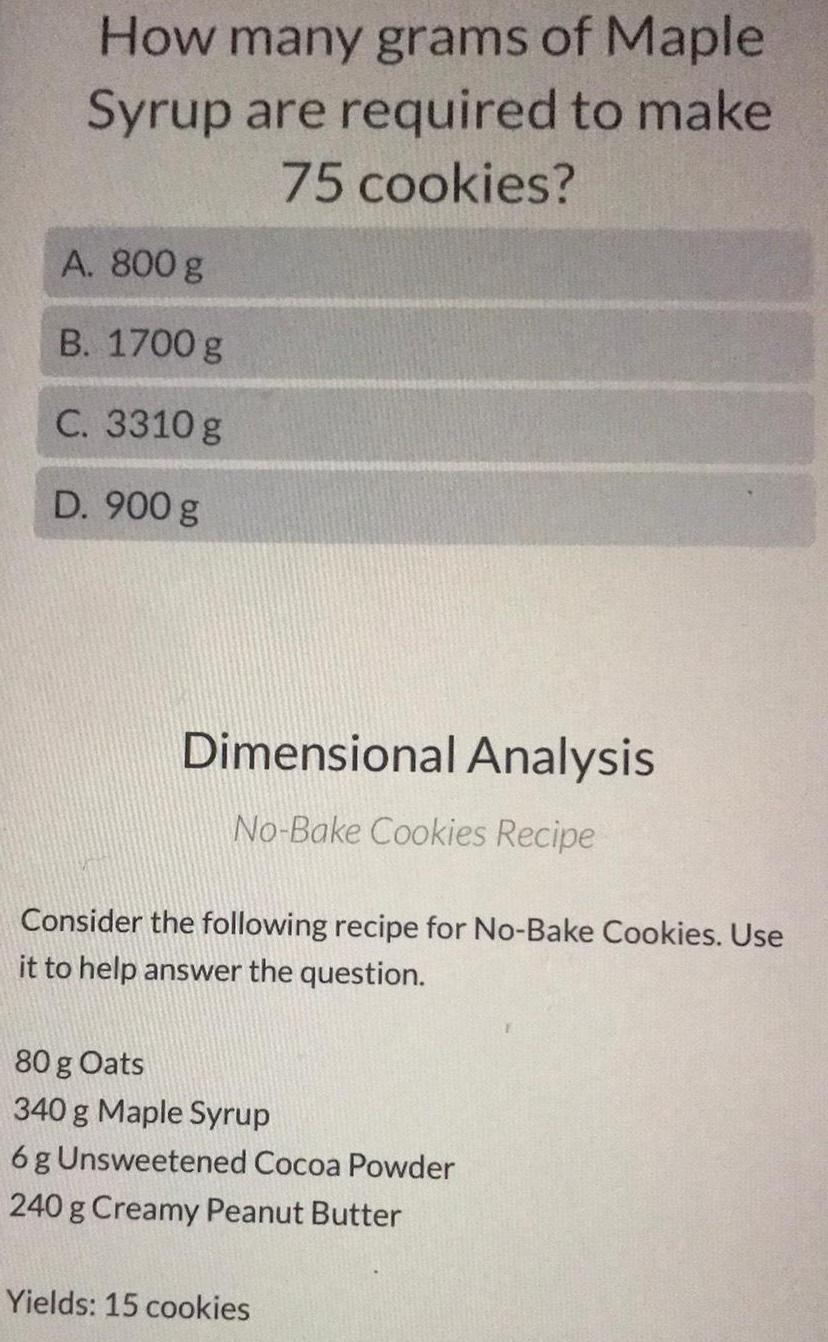Inorganic Chemistry
Coordination compounds
How many grams of Maple Syrup are required to make 75 cookies? A. 800 g B. 1700 g C. 3310 g D. 900 g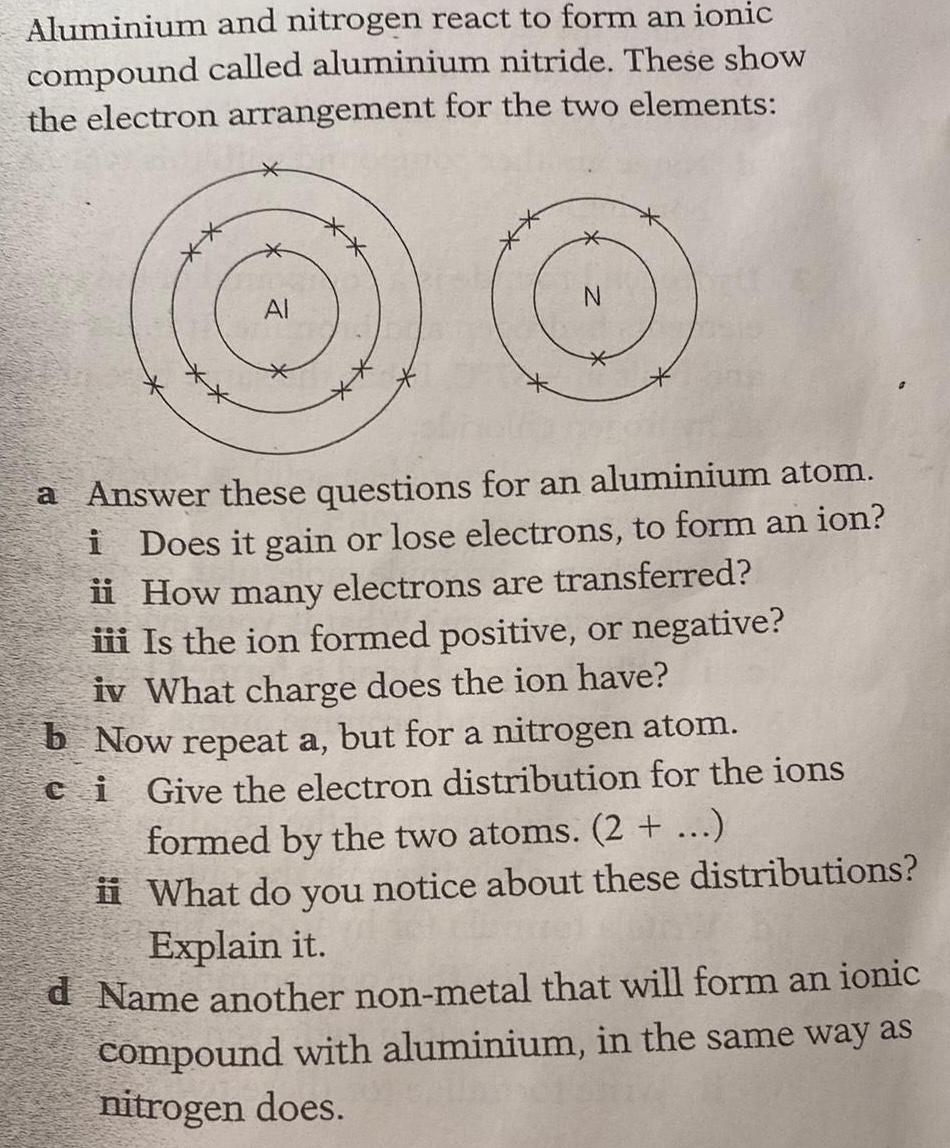Inorganic Chemistry
Classification of Elements and Periodicity
Aluminium and nitrogen react to form an ionic compound called aluminium nitride. These show the electron arrangement for the two elements: a. Answer these questions for an aluminium atom. i. Does it gain or lose electrons, to form an ion? ii. How many electrons are transferred? iii. Is the ion formed positive, or negative? iv. What charge does the ion have? b. Now repeat a, but for a nitrogen atom. c. Give the electron distribution for the ions formed by the two atoms. (2 + ...) ii. What do you notice about these distributions? Explain it. d. Name another non-metal that will form an ionic compound with aluminium, in the same way as nitrogen does.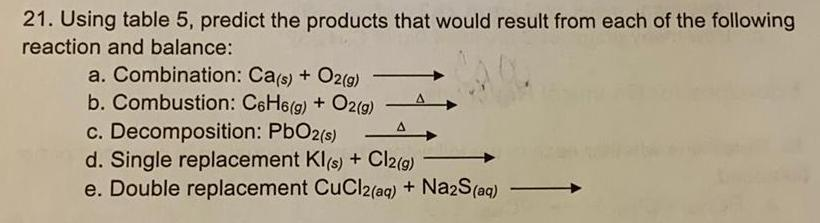Inorganic Chemistry
Preparation and Properties of Compounds
21. Using table 5, predict the products that would result from each of the following reaction and balance: a. Combination: Ca(s) + O2(g) -> b. Combustion: C6H6(g) + O2(g) -> c. Decomposition: PbO2 (s) -> d. Single replacement Kl(s) + Cl2(g) -> e. Double replacement CuCl2(aq) + Na2S (aq) ->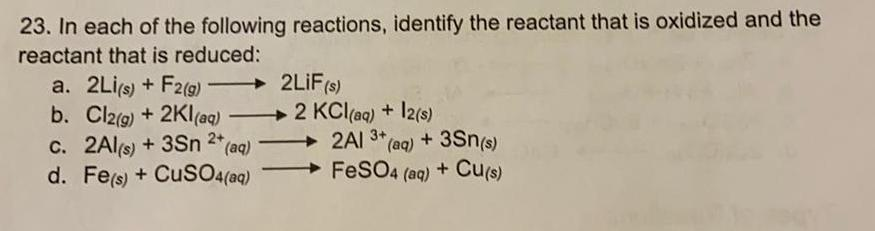Inorganic Chemistry
S Block - Group 2
In each of the following reactions, identify the reactant that is oxidized and the reactant that is reduced: a. 2Li(s) + F2 (g) → 2LIF(s) b. Cl2(g) + 2Kl(aq) →2 KCl(aq) + I2(s) c. 2Al(s) + 3Sn ²+ (aq) -> 2AI 3+ (aq) + 3Sn(s) d. Fe(s) + CuSO4(aq) → FeSO4 (aq) + Cu(s)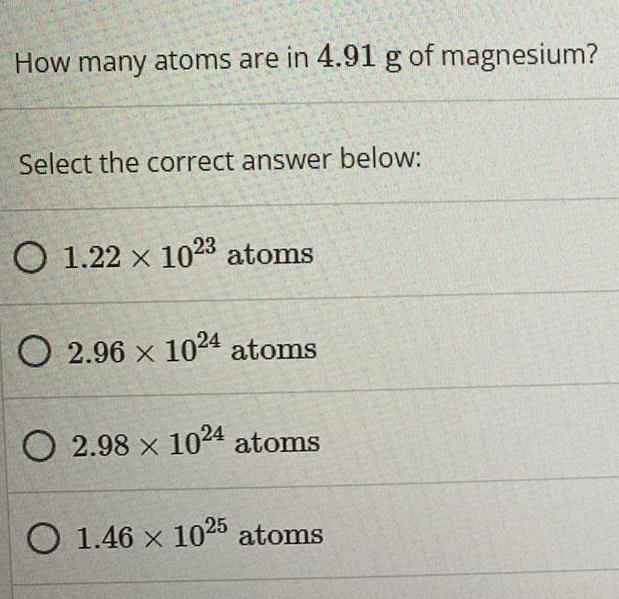Inorganic Chemistry
Classification of Elements and Periodicity
How many atoms are in 4.91 g of magnesium? Select the correct answer below: A. 1.22 x 10^23 atoms B. 2.96 x 10^24 atoms C. 2.98 x 10^24 atoms D. 1.46 x 10^25 atoms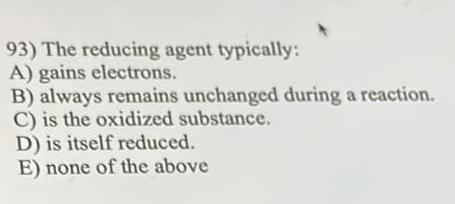Inorganic Chemistry
Preparation and Properties of Compounds
The reducing agent typically: A) gains electrons. B) always remains unchanged during a reaction. C) is the oxidized substance. D) is itself reduced. E) none of the above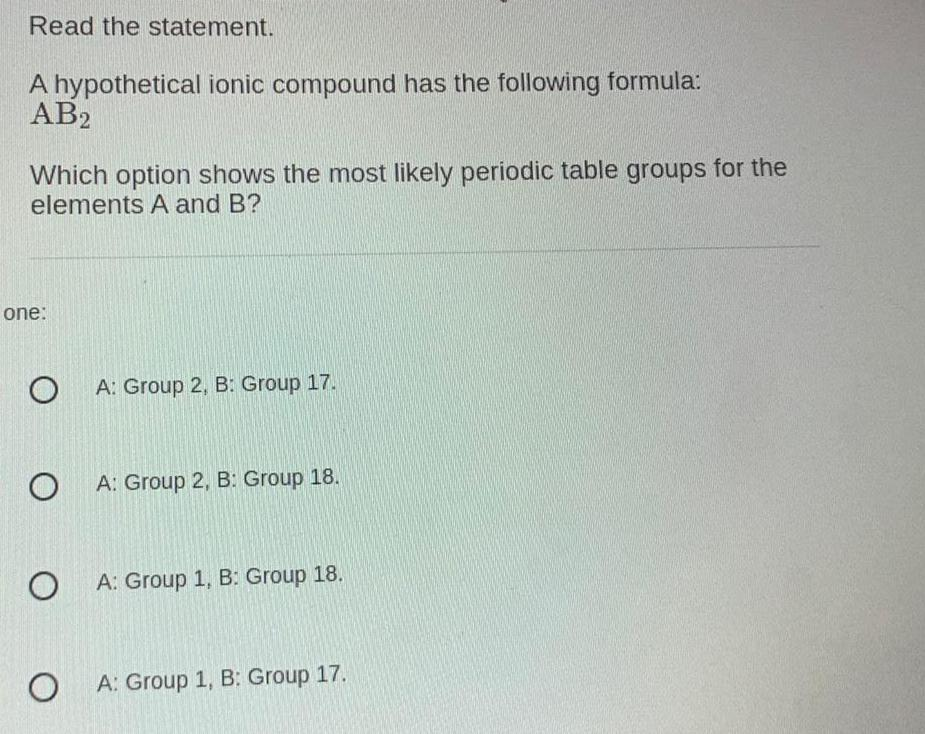Inorganic Chemistry
Classification of Elements and Periodicity
A hypothetical ionic compound has the following formula: AB2 Which option shows the most likely periodic table groups for the elements A and B? A. A: Group 2, B: Group 17. B. A: Group 2, B: Group 18. C. A: Group 1, B: Group 18. D. A: Group 1, B: Group 17.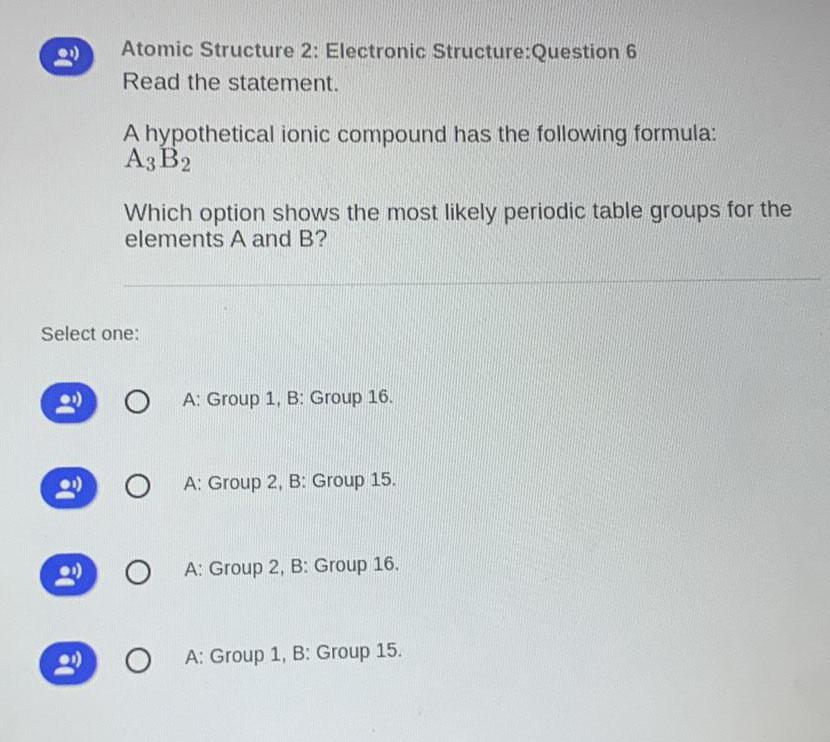Inorganic Chemistry
Classification of Elements and Periodicity
A hypothetical ionic compound has the following formula: A3 B2 Which option shows the most likely periodic table groups for the elements A and B? A. A: Group 1, B: Group 16. B. A: Group 2, B: Group 15. C. A: Group 2, B: Group 16. D. A: Group 1, B: Group 15.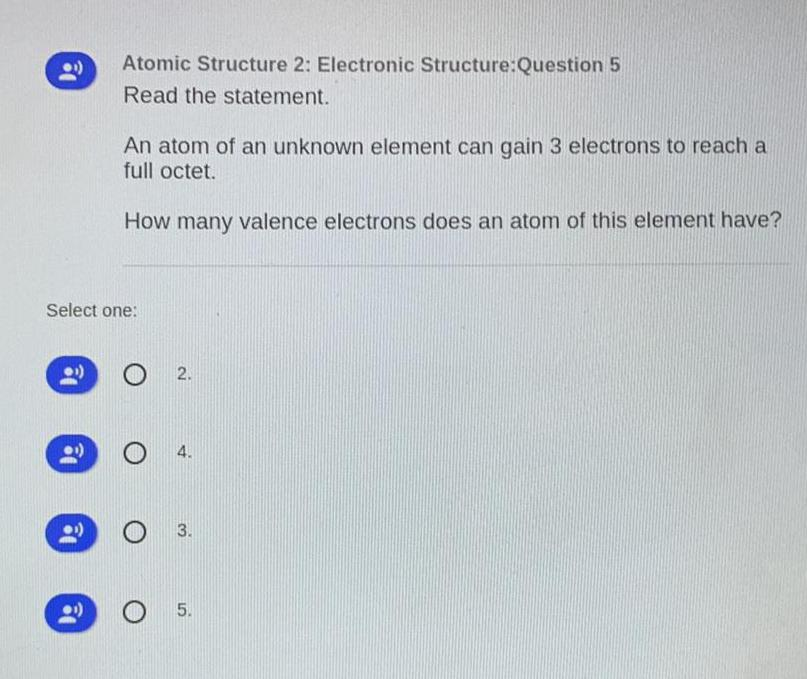Inorganic Chemistry
Classification of Elements and Periodicity
An atom of an unknown element can gain 3 electrons to reach a full octet. How many valence electrons does an atom of this element have? A. 2. B. 4. C. 3. D. 5.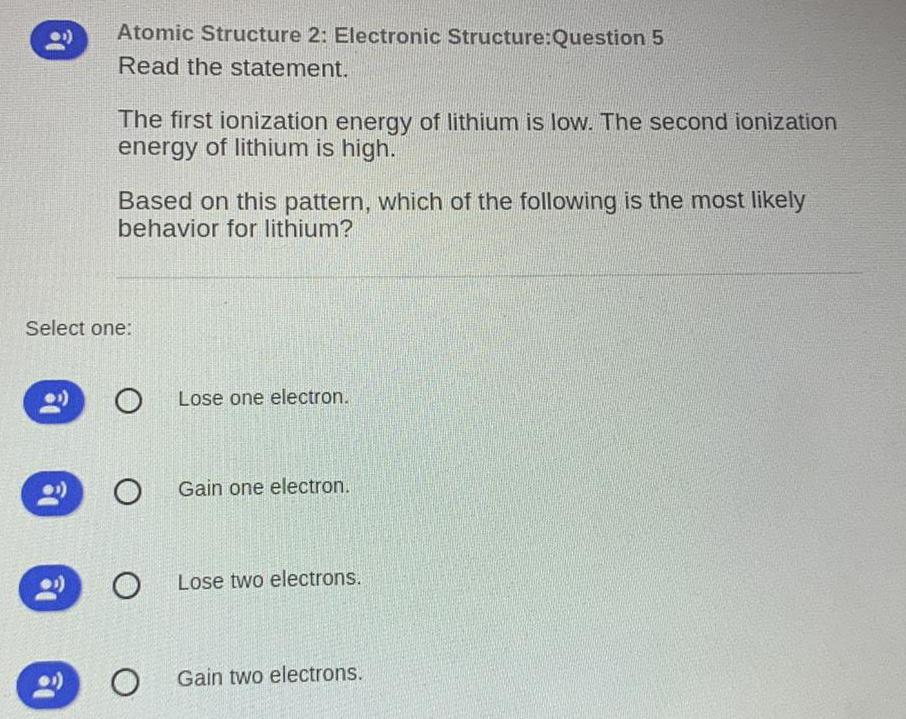Inorganic Chemistry
S Block - Group 1
The first ionization energy of lithium is low. The second ionization energy of lithium is high. Based on this pattern, which of the following is the most likely behavior for lithium? A. Lose one electron. B. Gain one electron. C. Lose two electrons. D. Gain two electrons.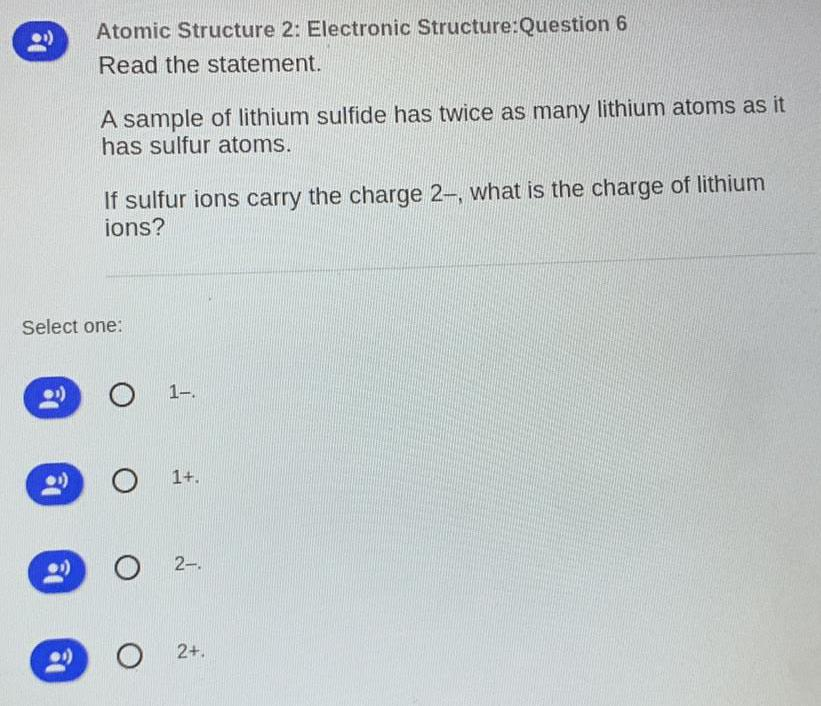Inorganic Chemistry
S Block - Group 2
A sample of lithium sulfide has twice as many lithium atoms as it has sulfur atoms. If sulfur ions carry the charge 2-, what is the charge of lithium ions? A. 1- B. 1+ C. 2- D. 2+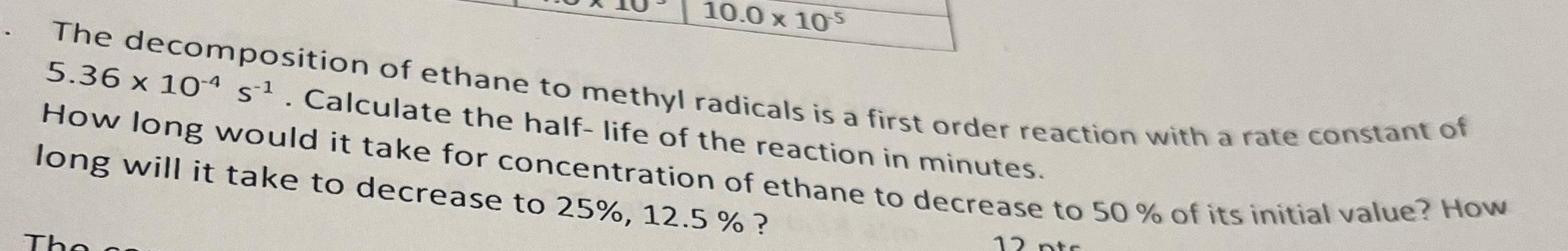Inorganic Chemistry
Qualitative analysis
The decomposition of ethane to methyl radicals is a first-order reaction with a rate constant of 5.36 x 104 s-¹. Calculate the half-life of the reaction in minutes. How long would it take for the concentration of ethane to decrease to 50% of its initial value? How long will it take to decrease to 25%, 12.5 %?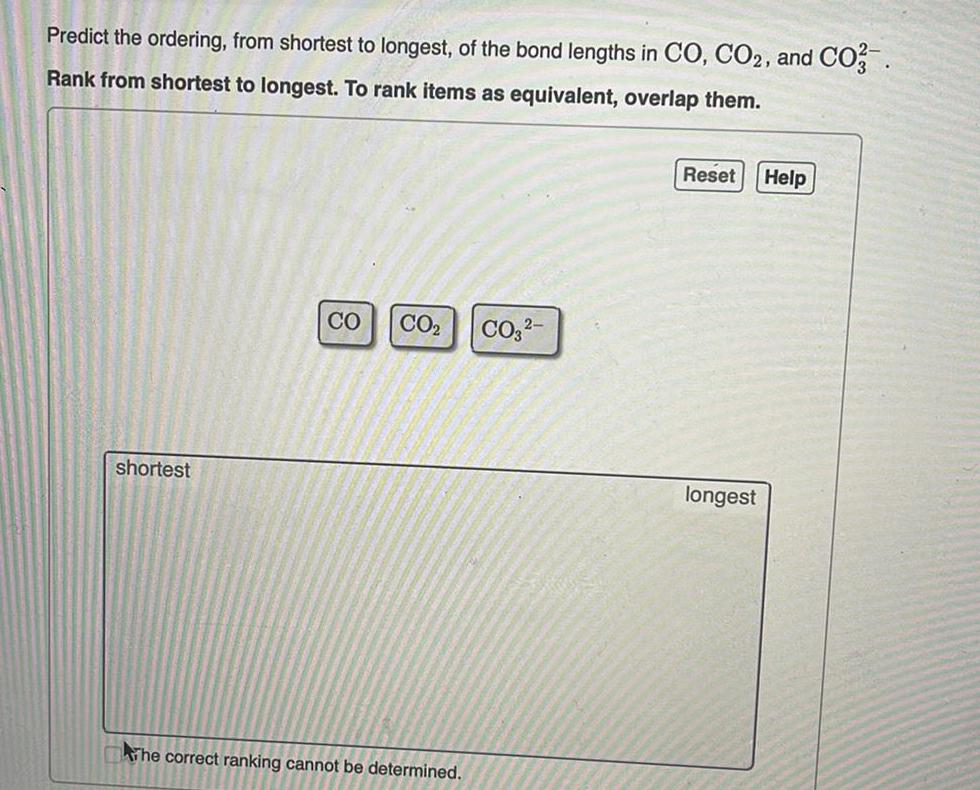Inorganic Chemistry
Classification of Elements and Periodicity
Predict the ordering, from shortest to longest, of the bond lengths in CO, CO2, and CO32- Rank from shortest to longest.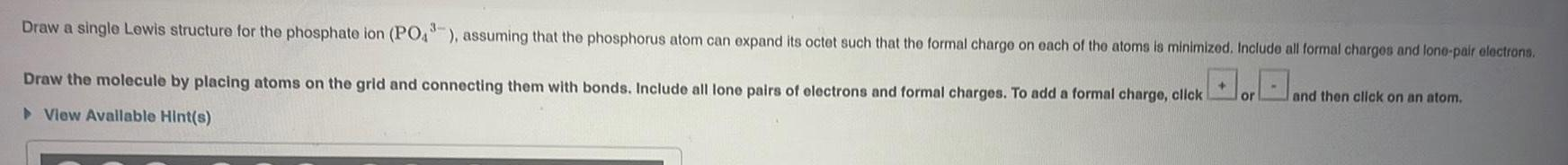Inorganic Chemistry
Classification of Elements and Periodicity
Draw a single Lewis structure for the phosphate ion (PO43), assuming that the phosphorus atom can expand its octet such that the formal charge on each of the atoms is minimized. Include all formal charges and lone-pair electrons.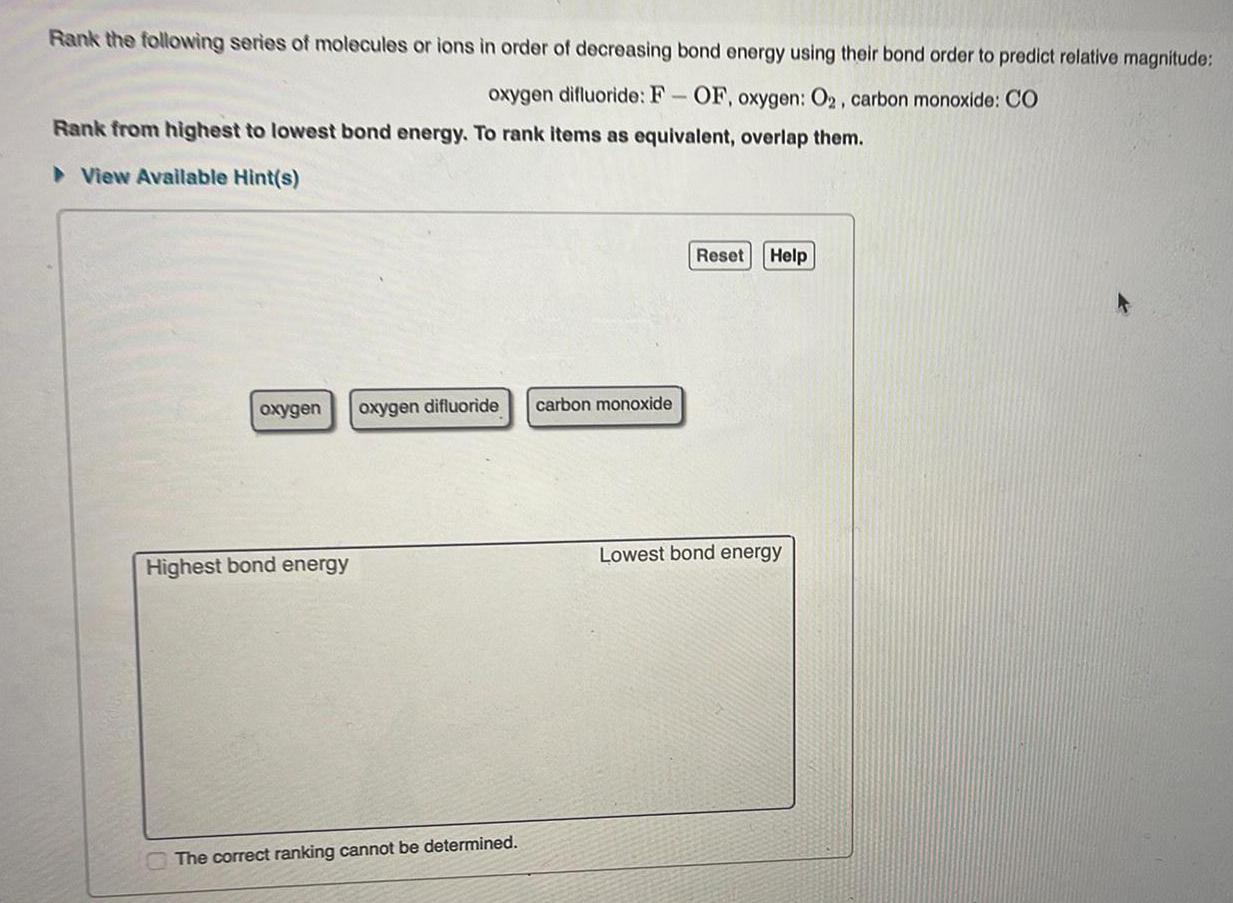Inorganic Chemistry
P Block - Group 16
Rank the following series of molecules or ions in order of decreasing bond energy using their bond order to predict relative magnitude: oxygen difluoride: F- OF, oxygen: O2, carbon monoxide: CO Rank from highest to lowest bond energy.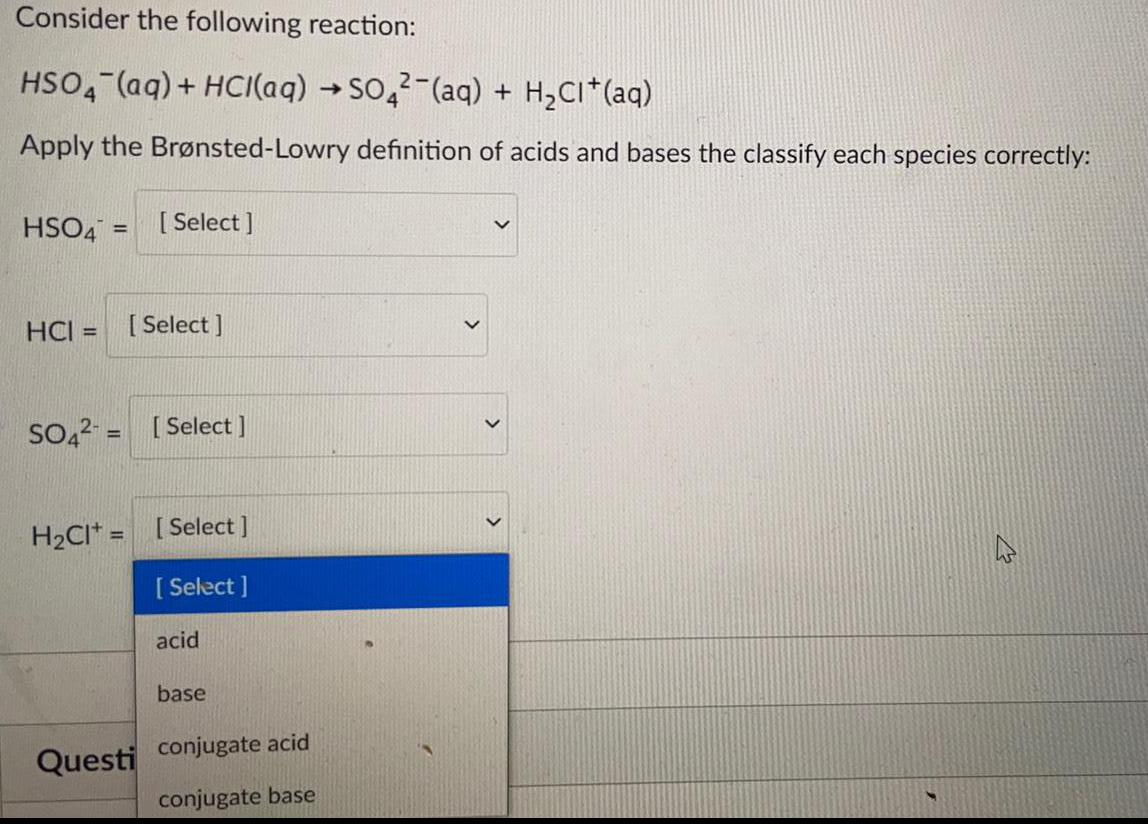Inorganic Chemistry
Qualitative analysis
Consider the following reaction: HSO4 (aq) + HCl(aq) → SO42-(aq) + H₂CI+ (aq) Apply the Brønsted-Lowry definition of acids and bases the classify each species correctly: HSO4 = HCI = SO4²- = H₂CI+=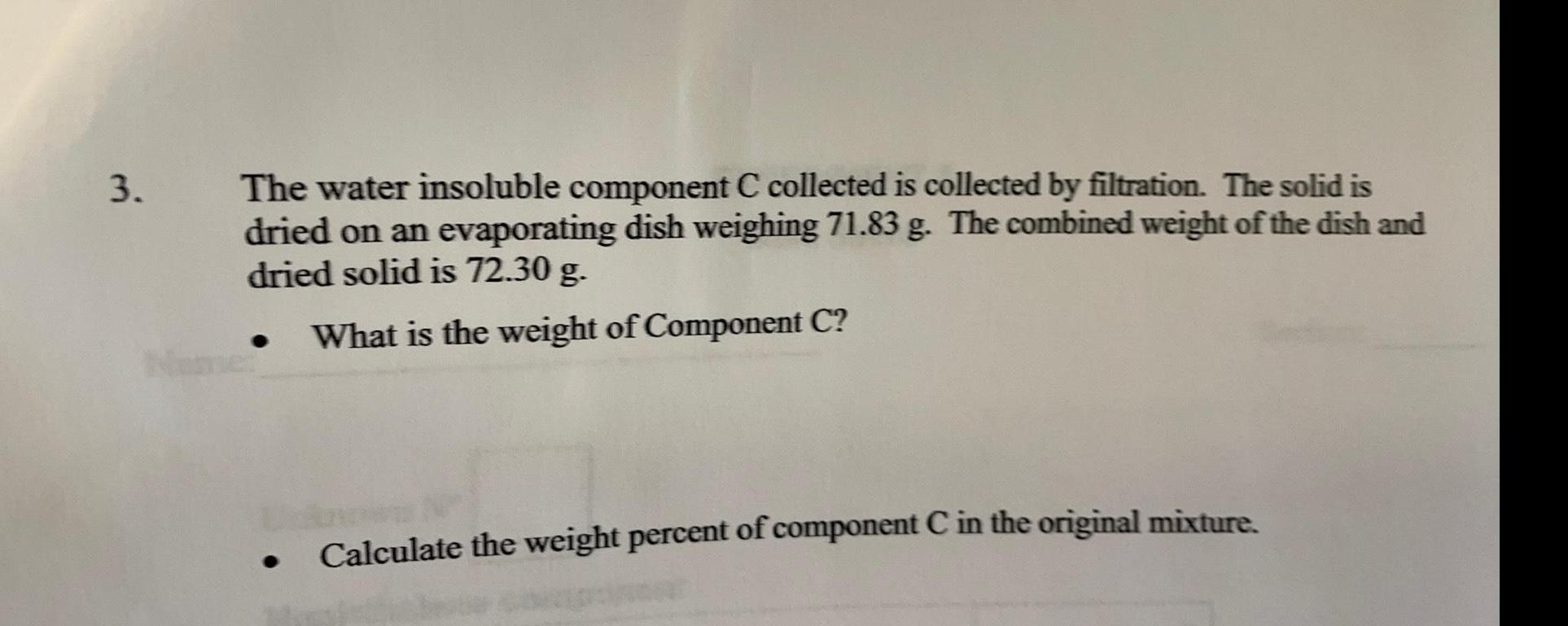Inorganic Chemistry
Qualitative analysis
The water-insoluble component C collected is collected by filtration. The solid is dried on an evaporating dish weighing 71.83 g. The combined weight of the dish and dried solid is 72.30 g. What is the weight of Component C? Calculate the weight percent of component C in the original mixture.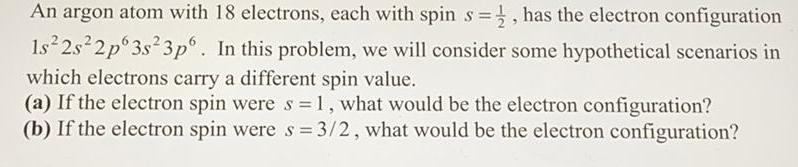Inorganic Chemistry
Preparation and Properties of Compounds
An argon atom with 18 electrons, each with spin s =1/2, has the electron configuration1s²2s²2p 3s²3p6. In this problem, we will consider some hypothetical scenarios in which electrons carry a different spin value. (a) If the electron spin were s = 1, what would be the electron configuration? (b) If the electron spin were s = 3/2, what would be the electron configuration?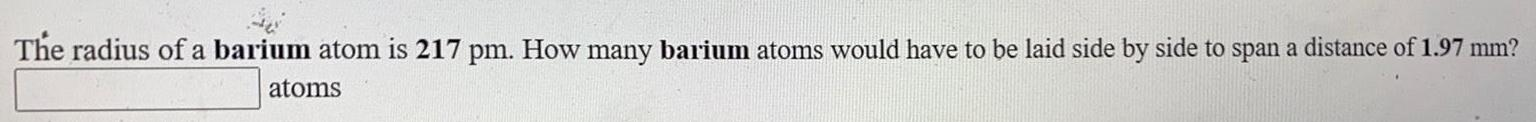Inorganic Chemistry
P Block - Group 13
The radius of a barium atom is 217 pm. How many barium atoms would have to be laid side by side to span a distance of 1.97 mm?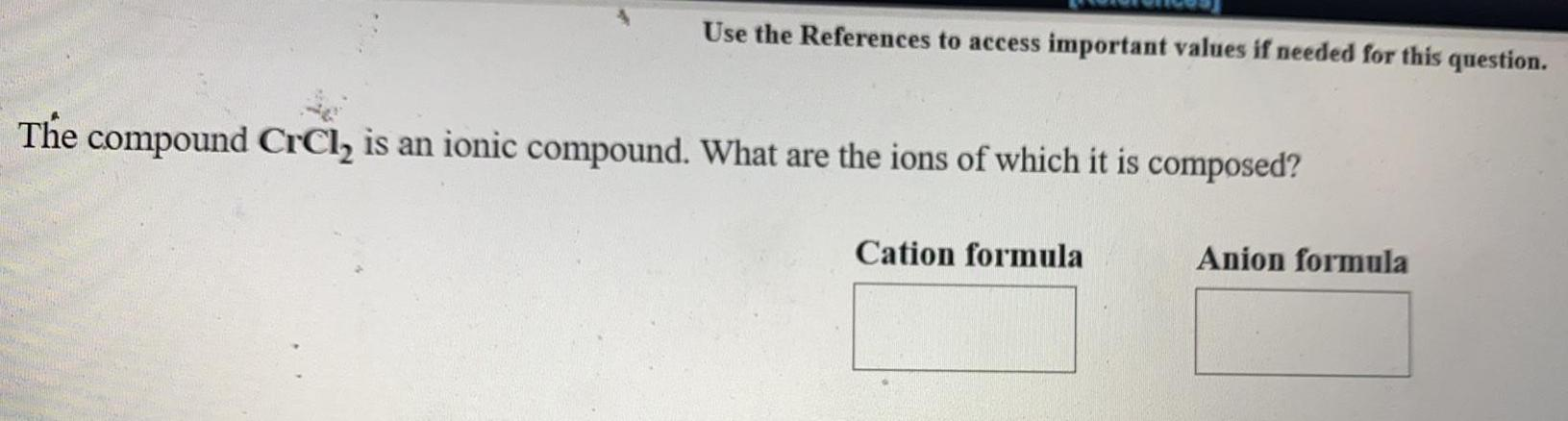Inorganic Chemistry
Preparation and Properties of Compounds
The compound CrCl₂ is an ionic compound. What are the ions of which it is composed? Cation formula ___ Anion formula ___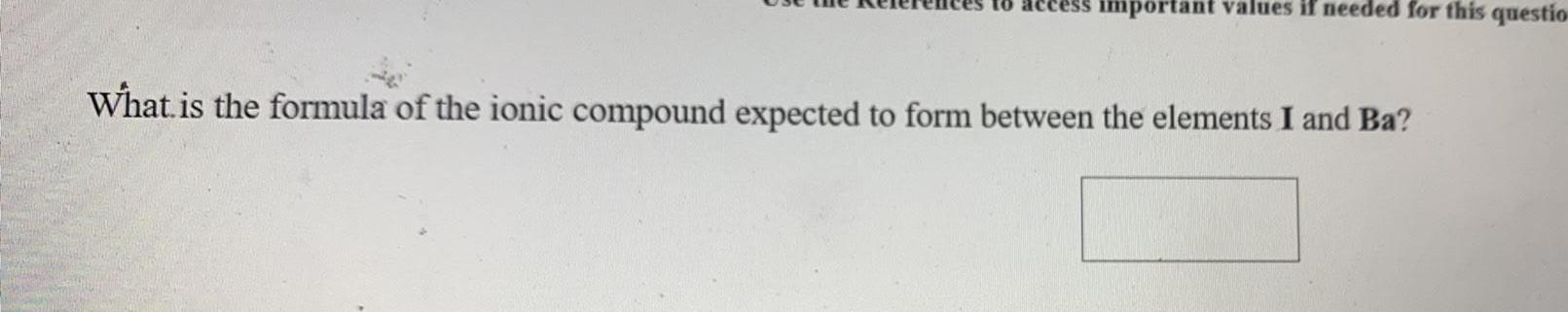Inorganic Chemistry
Preparation and Properties of Compounds
What is the formula of the ionic compound expected to form between the elements I and Ba?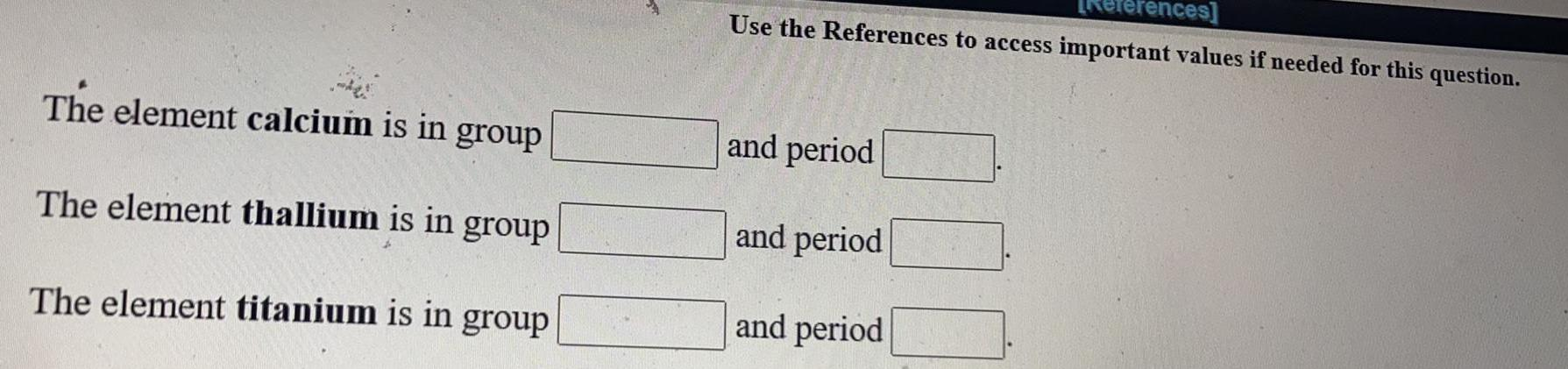Inorganic Chemistry
Classification of Elements and Periodicity
The element calcium is in group ___ and period ___ The element thallium is in group ___ and period ___ The element titanium is in group ___ and period ___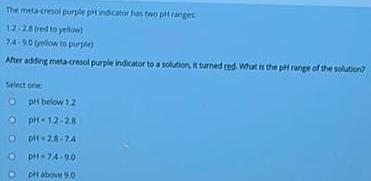Inorganic Chemistry
Qualitative analysis
The meta-cresol purple pt indicator has two pH ranges 12-28 (red to yellow) 7.4-9.0 (yellow to purple) After adding meta-cresol purple indicator to a solution, it turned red. What is the pH range of the solution? A. pH below 1.2 B. pH = 1.2 - 2.8 C. pH = 2.8 - 7.4 D. pH = 7.4 - 9.0 E. pH above 9.0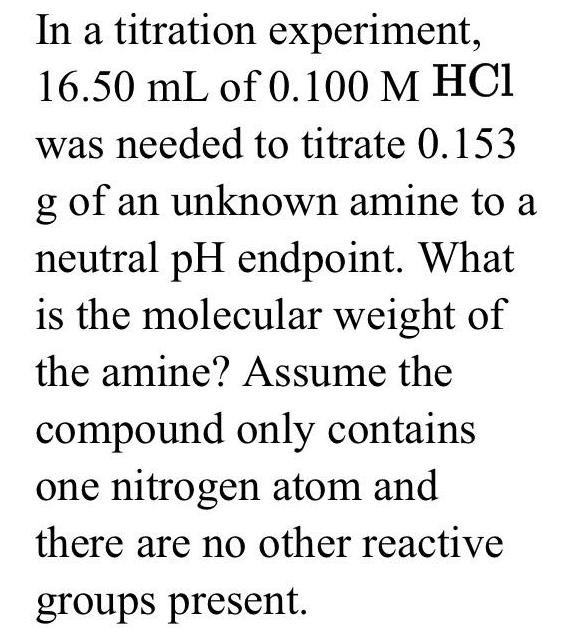Inorganic Chemistry
Qualitative analysis
In a titration experiment,16.50 mL of 0.100 M HCl was needed to titrate 0.153g of an unknown amine to a neutral pH endpoint. What is the molecular weight of the amine? Assume the compound only contains one nitrogen atom and there are no other reactive groups present.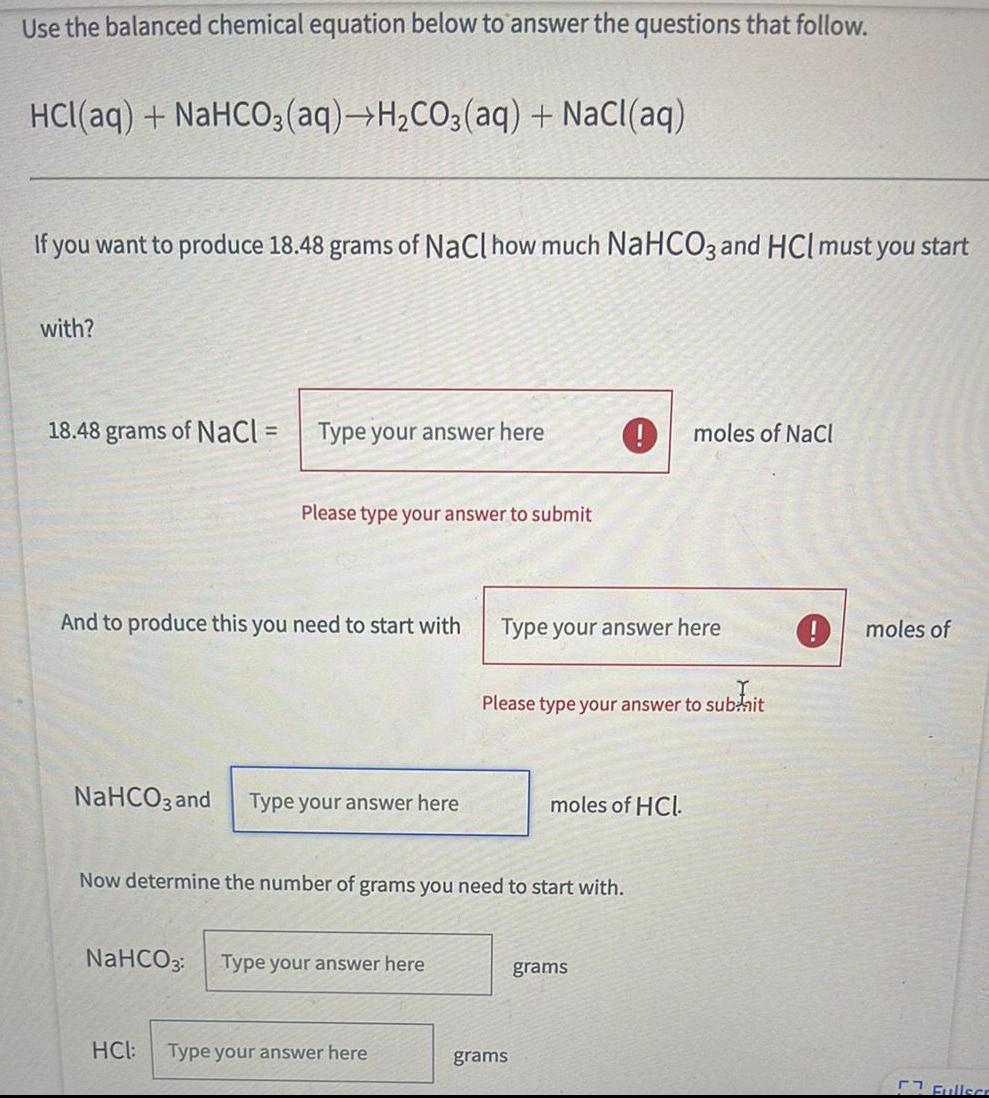Inorganic Chemistry
Preparation and Properties of Compounds
Use the balanced chemical equation below to answer the questions that follow. HCl(aq) + NaHCO3(aq) →H₂CO3(aq) + NaCl(aq) If you want to produce 18.48 grams of NaCl how much NaHCO3 and HCI must you start with? 18.48 grams of NaCl = And to produce this you need to start with ___ moles of NaHCO3 and ___ molrs of HCl. determine the number of grams you need to start with. NaHCO3 = ___ grams, HCl = ___ grams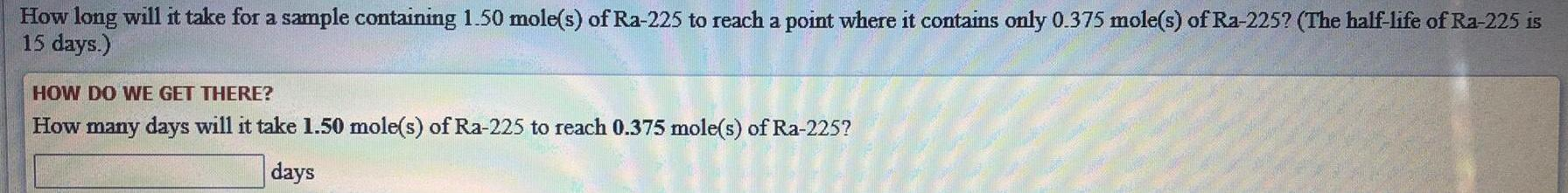Inorganic Chemistry
D Block elements
How long will it take for a sample containing 1.50 mole(s) of Ra-225 to reach a point where it contains only 0.375 mole(s) of Ra-225? (The half-life of Ra-225 is15 days.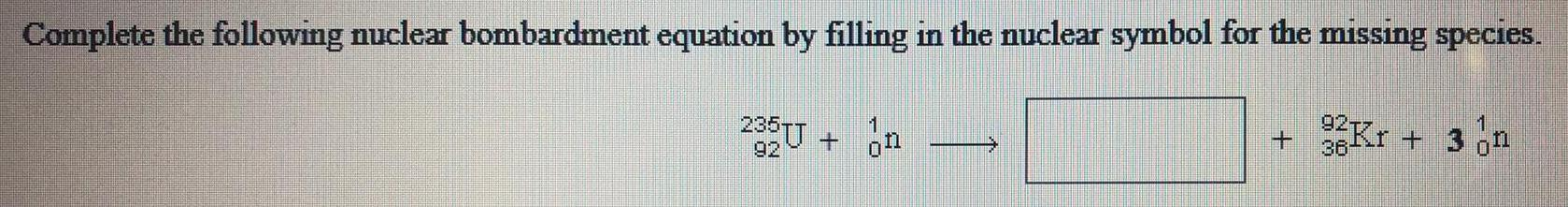Inorganic Chemistry
Preparation and Properties of Compounds
Complete the following nuclear bombardment equation by filling in the nuclear symbol for the missing species. 92U235 + 0n1 -> ___ + 36Kr92 + 3 0n1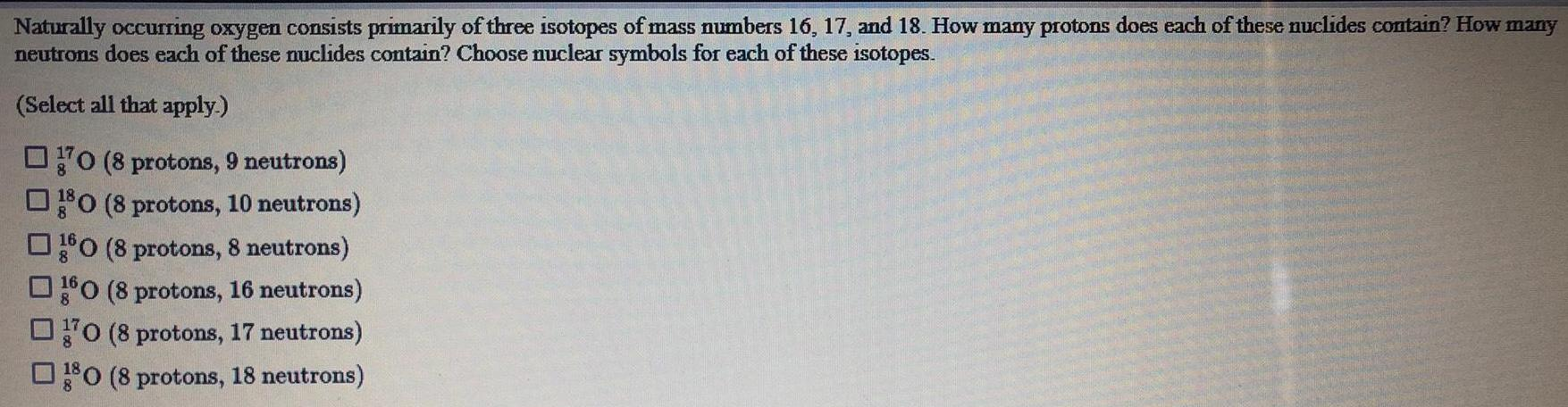Inorganic Chemistry
S Block - Group 1
Naturally occurring oxygen consists primarily of three isotopes of mass numbers 16, 17, and 18. How many protons does each of these nuclides contain? How many neutrons does each of these nuclides contain? Choose nuclear symbols for each of these isotopes. (Select all that apply.) A. 8O17 (8 protons, 9 neutrons) B. 8O18 (8 protons, 10 neutrons) C. 8O16 (8 protons, 8 neutrons) D. 8O17 (8 protons, 16 neutrons) E. 8O18 (8 protons, 18 neutrons)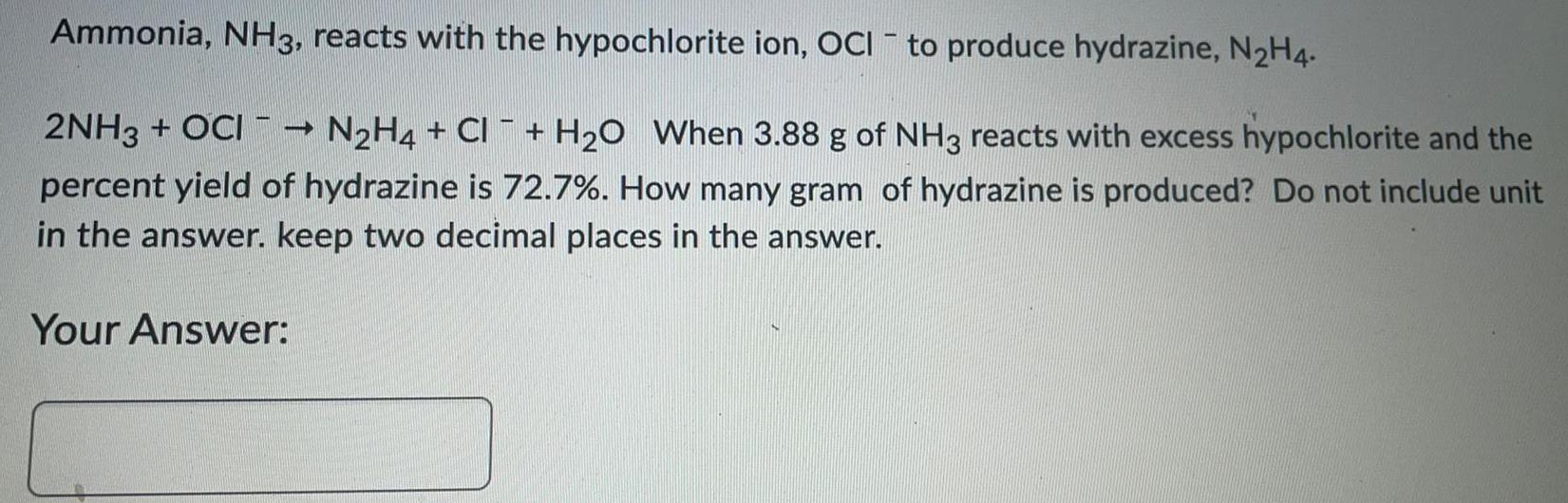Inorganic Chemistry
P Block - Group 14
Ammonia, NH3, reacts with the hypochlorite ion, OCI- to produce hydrazine, N2H4. 2NH3 + OCI- → N2H4 + CI ¯ + H₂O When 3.88 g of NH3 reacts with excess hypochlorite and the percent yield of hydrazine is 72.7%. How many gram of hydrazine is produced?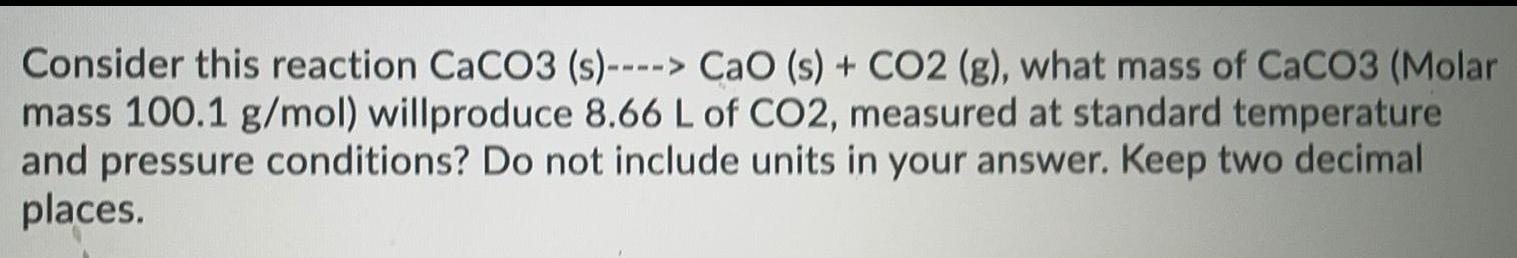Inorganic Chemistry
Preparation and Properties of Compounds
Consider this reaction CaCO3 (s)---> CaO (s) + CO2 (g), what mass of CaCO3 (Molar mass 100.1 g/mol) will produce 8.66 L of CO2, measured at standard temperature and pressure conditions?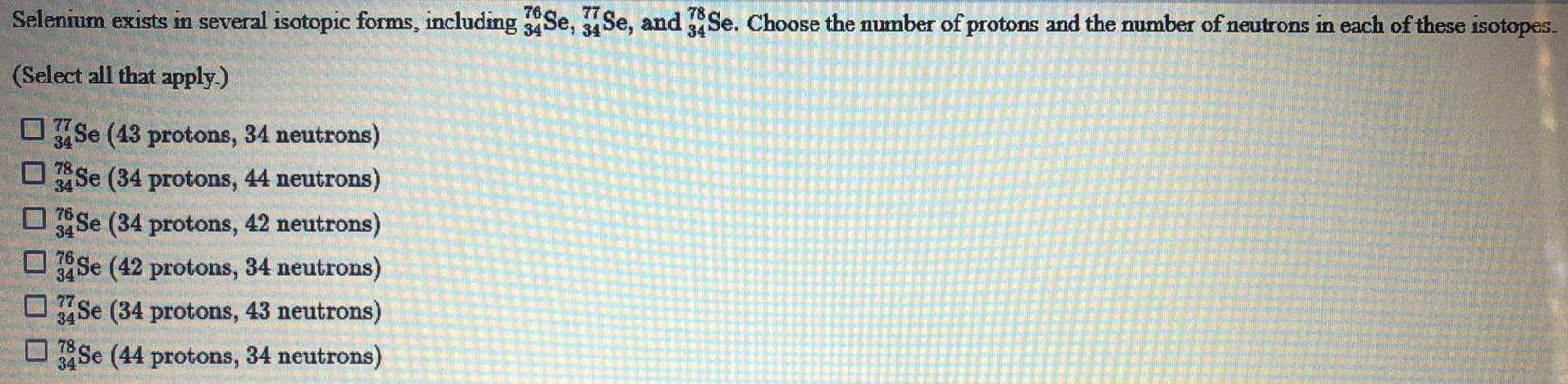Inorganic Chemistry
Classification of Elements and Periodicity
Selenium exists in several isotopic forms, including 34Se76, 34Se77, and 34Se78. Choose the number of protons and the number of neutrons in each of these isotopes. (Select all that apply.) 34Se77 (43 protons, 34 neutrons) 34Se78 (34 protons, 44 neutrons) 34Se76 (34 protons, 42 neutrons) 34Se76 (42 protons, 34 neutrons) 34Se77 (34 protons, 43 neutrons) 34Se78 (44 protons, 34 neutrons)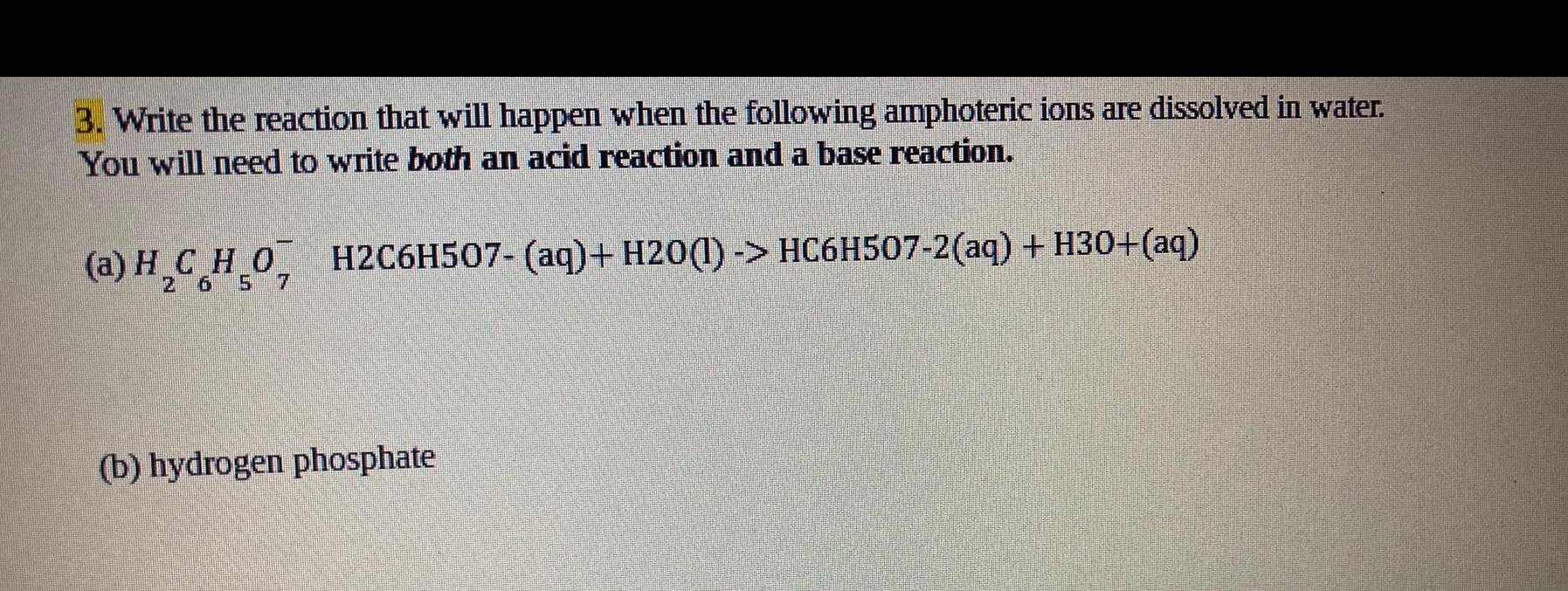Inorganic Chemistry
Preparation and Properties of Compounds
Write the reaction that will happen when the following amphoteric ions are dissolved in water. You will need to write both an acid reaction and a base reaction. (a) H2C6H5O7- H2C6H507- (aq)+ H2O(l) -> HC6H507-2(aq) + H30+(aq) (b) hydrogen phosphate